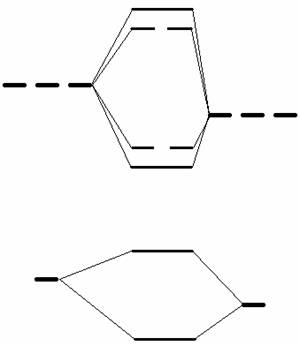Practice midterm questions for Chem 101 Section J1, Fall 2004

1.         Supply the missing name or formula:

strontium nitrate hydrate            Sr(NO3)2 ∙ H2O

dinitrogen tetroxide       N2O4

iron (III) perchlorate     Fe(ClO4)3

mercury (I) chlorate            Hg2(ClO3)2

thallium acetate              TlCH3COO

hypobromous acid             HBrO(aq

2.         Answer the following by filling in each blank with the appropriate answer, or by circling the correct choice, as appropriate.

a)         Which is the stronger oxidizing agent, Pb(IV) or Pb(II)?            Pb(IV)

b)         Which element has a larger sixth ionization energy, P or S? P

c)         The bonding within the compound SbF5 is primarily ionic / covalent? (Circle one)

d)         The electron affinity of groups 2 and 18 is close to zero.  Which other group (in the s and p blocks) has an electron affinity which is smaller than would be expected on the basis of the general trends?   15

f)          Identify the neutral element that is isoelectronic with the cation Po4+.  Hg

g)         Which atom has the greater Zeff, Si or S? S

h)         Which ion has the largest radius, K+, Cl-, Sc3+ or

S2-?  S2-

i)          Arrange the following atoms in order of decreasing atomic radius:

In, Te, Sn, Kr In Sn Te Kr

j)          A new element is discovered.  It has two isotopes.  The relative abundances of the isotopes and their masses are 18 % isotope 1, mass 350.0 g/mol and isotope 2, mass 352.0 g/mol.  What is the atomic mass of the element?

a)         351.6

b)         351.0

c)         350.4

d)         352.0

e)         350.0

k)         The oxidation state of vanadium in VO2+ is :

a)         -2

b)         +2

c)         +1

d)         -4

e)         +4

l)          The oxidation state of boron in B5H9 is:

a)         +3

b)         0

c)         -1/2

d)         + 9/5

e)         - 9/5

m)        Of all the known elements in the periodic table, the atom with the largest radius is: Fr

n)         As we go down a particular group in the periodic table, the effective nuclear charge (increases/decreases/stays the same) ___________________

o)         Identify the neutral element that is isoelectronic with the cation Po4+ ________

p)   Provide the appropriate value for the missing quantum number:
n=1, l= 0, ml= 0 ms=1/2

q)   Which of the following isoelectronic species is largest: Rb+, Kr, Br-, Se2-? Se2-

d)      How many electrons can there be with ml = 0 when n=5?

10

3.     Write down the complete electronic configuration for each of the following ions and classify each as a noble gas ion, a pseudo-noble gas ion or an inert pair ion.

Refer to page A17 in the text for electronic configurations of the elements.  The answer I have given is the element that the ion is isoelectronic with.

i)              As3-  isoelectronic with Kr

ii)      Cd2+ isoelectronic with Pd

iii)      Cs-  isoelectronic with Ba

iv)     Sn2+ isoelectronic with Cd

v)      Tl3+ isoelectronic with Hg

4.         a) What trication has the configuration [Xe]6s24f145d10?

This is some ion of the form M3+.  When it’s a neutral atom, it must have three more electrons than the configuration shown.  That would make it the atom with the configuration [Xe]6s24f145d106p3.

b) Is this ion paramagnetic or diamagnetic?  Why or why not?

Diamagnetic.  All its electrons are paired.

c) Classify this ion according to the type of electronic configuration.

M3+ is an inert pair cation.

5.         The electron affinity for nitrogen is very small, whereas for oxygen and carbon it is larger.  Explain this observation.

The configuration for N is [He]2s22p3.  Each p orbital is half occupied, so that adding an electron would necessitate that the electron be paired with another electron.  These paired electrons would repel each other, reducing the amount of energy released when an electron is acquired (the electron affinity, in other words) to nearly zero.

When C or O gain an electron it goes into an empty orbital, so there isn’t any repulsion due to paired electrons in the p subshell.

6.  Complete the following table:

Molecular             Lewis Dot          Sketch of the                      Molecular                     Is the

Species                 Structure            Molecule (with                   Shape                           species

lone pairs)                                                            polar?

SeF                            These are all in the textbook.

SbCl5

PF6-

7.                     Draw three valid resonance structures for fulminic acid.

Show all non-zero formal charges.

Pick the structure(s) that most accurately depict(s) the bonding in the molecule.

(Note: The sequence of atoms in the molecule is CNOH).

8.         The empty molecular orbital diagram for NO- is given below. Complete the molecular orbital diagram and then answer the questions posed below.    Be sure to identify Atoms A and B and label all of the molecular orbitals in the centre of the diagram.

i)        What is the bond order for NO- in the ground state?

ii)       What is the total number of p-bonds in NO-?

iv)     Consider NO+.  Compare its internuclear separation to that of NO-.Atom A                                            Atom B